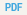Staff Reports
Revisiting Useful Approaches to Data-Rich Macroeconomic Forecasting
 May 2008 Number 327 Revised October 2015 JEL classification: C22, C53, E37, E47

Authors: Jan J. J. Groen and George Kapetanios

This paper analyzes the properties of a number of data-rich methods that are widely used in macroeconomic forecasting, in particular principal components (PC) and Bayesian regressions, as well as a lesser-known alternative, partial least squares (PLS) regression. In the latter method, linear, orthogonal combinations of a large number of predictor variables are constructed such that the covariance between a target variable and these common components is maximized. Existing studies have focused on modelling the target variable as a function of a finite set of unobserved common factors that underlies a large set of predictor variables, but here it is assumed that this target variable depends directly on the whole set of predictor variables. Given this setup, it is shown theoretically that under a variety of different unobserved factor structures, PLS and Bayesian regressions provide asymptotically the best fit for the target variable of interest. This includes the case of an asymptotically weak factor structure for the predictor variables, for which it is known that PC regression becomes inconsistent. Monte Carlo experiments confirm that PLS regression is close to Bayesian regression when the data has a factor structure. When the factor structure in the data becomes weak, PLS and Bayesian regressions outperform principal components. Finally, PLS, principal components, and Bayesian regressions are applied on a large panel of monthly U.S. macroeconomic data to forecast key variables across different subperiods, and PLS and Bayesian regression usually have the best out-of-sample performances.

 Available only in PDF52 pages / 408 kb
By continuing to use our site, you agree to our Terms of Use and Privacy Statement. You can learn more about how we use cookies by reviewing our Privacy Statement.